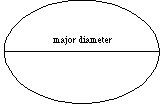index: click on a letter A B C D E F G H I J K L M N O P Q R S T U V W X Y Z A to Z index index: subject areas numbers & symbols sets, logic, proofs geometry algebra trigonometry advanced algebra & pre-calculus calculus advanced topics probability & statistics real world applications multimedia entrieswww.mathwords.com about mathwords website feedback

 Major Diameter of an Ellipse The segment joining the vertices of an ellipse, or the length of that segment. The major diameter passes through the center and foci. It is an ellipse's longest diameter.See also